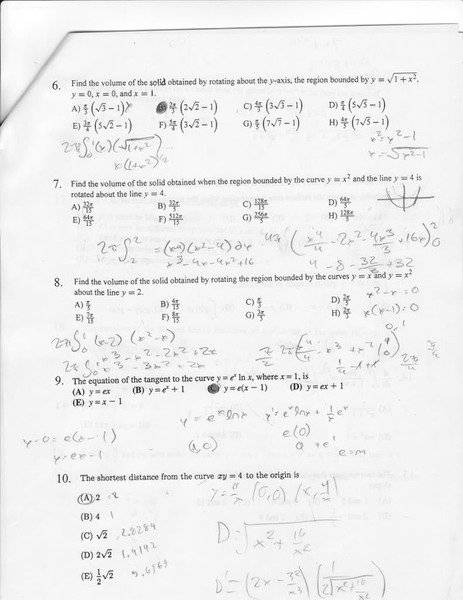# Volume of a solid

Thanks to the awesome help on this board, I am narrowing my problems down quickly. I have already answered problems #6, 7, 9, and 10 as b, f, c, and a respectively. I wouldn't mind if you checked them but it's not my biggest worry. Instead I have given many attempts at number 8 using the disc/washer and shell formula. The page is out of date because I have been working on it since that page has been scanned and I no longer have access to that scanner. Any clarification on the formulas and their uses in this problem would be great.## Answers and Replies

HallsofIvy
Science Advisor
Homework Helper
Problem 8 is "Find the volume of the solid obtained by rotating the region bounded by the curves y= x and y= x2 around the line y= 2.

First, of course, draw a picture. You should see that vertical line drawn from y= 2 will cross that region first at y= x and then at y= x2. That means that a "washer" formed by rotating the figure about y= 2 will have inner radius 2- x and outer radius 2- x2. The areas of the two circles with those radii will be $\pi(2- x)^2$ and $\pi(2- x^2)^2$. The area of the "washer" between them will have area equal to the difference of those. Integrate that with respect to dx from x= 0 to x= 1.

If you want to do it by "shells", each shell will be a thin cylinder of thickness dy, radius 2- y, and height $\sqrt{y}- y$. The surface area of that will be $2\pi(2- y)(\sqrt{y}- y)$ and the volume will be $2\pi(2- y)(\sqrt{y}- y)dy$ integrated from y= 0 to y= 1.